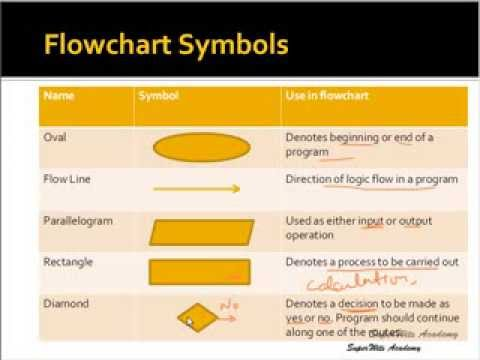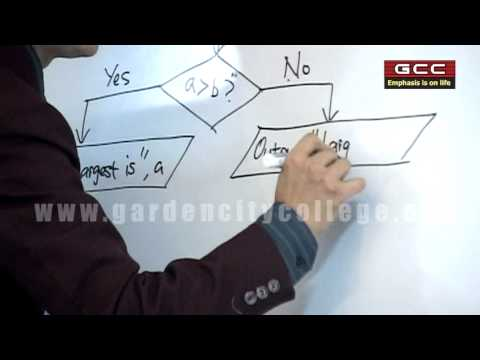## Program Designing Tools

Subject: Computer

#### Overview

A set of rules that define how a particular problem can be solved in finite number of steps is known as algorithm. It is step by step representation of instructions to solve the problem. This note provides an information about different types of program designing tools.
##### Program Designing ToolsWe know that computer do not have its own thinking power and working capacity. So, it is the user depended on the machine. All the activities of a computer system are controlled and processed by the instructions and information provided by the user. The instructions which we provide to the computer is known as commands. Collection of instructions and data in a systematic form to solve the problems is called program.

We have to make some systematic plan of action to solve the problem using a computer. Such plan of action can be represented in systematic order using different tools and technique. Popular tools and technique used to represent the programs are as follows:

• Algorithm
• Flowchart
• Pseudo code

#### Algorithm

A set of rules that define how a particular problem can be solved in finite number of steps is known as algorithm. It steps by step representation of instructions to solve the problem. Algorithm must be composed of the finite set of steps.A good algorithm should have following features:

• Input: specified and required input values.
• Output: outcome values or solution of the problem
• Definite: it must be clearly defined what should be done
• Effective: it must be able to perform each step exactly in a finite amount of time.
• Finite: it must have finite number of steps or operations.
• Correct: it must be able to generate correct and desirable output.

• Algorithm helps to write the program.
• It makes us easy to understand about facts of the program.
• It helps to change the program according to our needs.

• It takes long time to write the program.
• Languages used in the algorithm may not be convenient.

Example:

Step 1: Start

Step 2: Input Amount, P

Step 3: Input Time, T

Step 4: Input Rate, R

Step 5: Calculate Interest, I =(P*T*R)/100

Step 6: Print Interest, I

Step 7: Stop

#### Flowchart

A flowchart is the pictorial representation of step by step solution to a problem. It is one of the oldest technique in programming. A flowchart is independent of the programming language like algorithm. It helps to understand the program more easily. There are two types of the flowchart. They are:

• System flowchart
• Program flowchart

Rules of developing flowchart

• Analyse the input, process, storage and output of the program.
• Use standard symbols and arrowhead to the direction of flow of data and instructions.
• We should use a simple word which can be easily understood by other programmers.
• There should be the list of activities inside each symbol.
• It should express all sections like input, process, logical and output.
• it should not be any programming language oriented.• It is an easy method of communication to represent the program.
• It is program independent so applicable for all types of programming languages.
• It represents all components of the program like input, process, logic and output.
• It is easier to understand.

• It cannot replace all types of logic used by the computer program.
• It takes long time to represent all module of the computer program.
• It is not suitable and complex for the big program.

#### Pseudo code

"Pseudo" means duplicate and "code" means direction. Pseudo code is an outline of a program written in a form that can easily be converted into real programming statements. The pseudo code cannot be compiled nor executed and there are no real formatting or syntax rules. It is simply one step - an important one - in producing the final code. The benefit of pseudocode is that it enables the programmer to concentrate on the algorithms without worrying about all the syntactic details of a particular programming language.

##### Things to remember
• Popular tools and technique used to represent the programs are Algorithm, Flowchart, and Pseudocode.
• A set of rules that define how a particular problem can be solved in finite number of steps is known as algorithm.
• Algorithm helps to write a program.
• Flowchart is a pictorial representation of step by step solution of a problem. It is one of the oldest technique in programming.
• Pseudo code is an outline of a program written in a form that can easily be converted into real programming statements.
• It includes every relationship which established among the people.
• There can be more than one community in a society. Community smaller than society.
• It is a network of social relationships which cannot see or touched.
• common interests and common objectives are not necessary for society.
##### Videos for Program Designing Tools##### Computer, Algorithm and Flowchart##### Concepts of Algorithm, Flow Chart & C Programming

Collection of instructions and data in a systematic form to solve the problems is called program.Popular tools and technique used to represent the programs are as follows:

• Algorithm
• Flowchart
• Pseudo code

A set of rules that define how a particular problem can be solved in finite number of steps is known as algorithm.

• Algorithm helps to write a program.
• It makes us easy to understand about facts of a program.
• It helps to change the program according to our needs.

Flowchart is a pictorial representation of step by step solution to a problem. It is one of the oldest technique in programming.

• It is the easy method of communication to represent the program.
• It is program independent so applicable for all types of programming languages.
• It represents all components of the program like input, process, logic and output.

A good algorithm should have following features:

• Input: specified and required input values.
• Output: outcome values or solution of the problem
• Definite: it must be clearly defined what should be done
• Effective: it must be able to perform each step exactly in a finite amount of time.
• Finite: it must have finite number of steps or operations.
• Correct: it must be able to generate correct and desirable output.

Pseudo code is an outline of a program written in a form that can easily be converted into real programming statements which cannot be compiled nor executed and there are no real formatting or syntax rules.